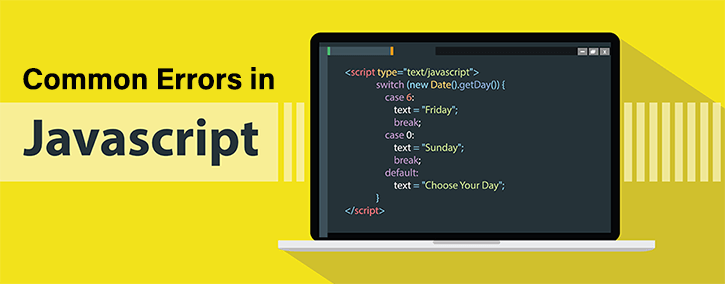Common Errors in JavaScript || JavaScript Tutorials# Common errors in JavaScript

2557

"Coding error can never become a big mistake unless a developer declines to make its correction"

Errors are part of learning. As a human being, mistakes are natural. Every coding whether it is of scripting language or programming, the developer may suffer from errors during program execution. These errors can cause delay or unexpected results. To prevent these errors and for time savage, coding tag has prepared this article to cover major errors that JavaScript developers are required to be aware of.This post would be beneficial for the beginner of JavaScript as there are great chances of mistake occurrences while coding. They can also refer to online JavaScript tutorials for beginners for rapid learning.

This article has been prepared after summarizing real-time errors of JavaScript occurred by developers in various web-projects. The following are a list of common errors in JavaScript along with their solutions that can be prevented from happening again or debugging them.

1. Improper function definitions in looping:

```function bible(x) {
var j = 0;
while (j < 8) {
if (j == 2) {
break;
}
j += 2;
} return j * x;
}
```

In this program, x is not defined properly

Solution

```function bible(x) {
var j = 0;
var x=4;
while (j < 8) {
if (j == 2) {
break;
}
j += 2;
} return j * x;
}```

2. Missing curly bracket inside function code:

```var book = book1() {
if (bible) {
} else {
editBibles();
};```

Solution:

```var book = book1() {
if (bible) {
} else {
editBibles();
}};```

3. TypeError: 'undefined' object:

```function monika(y) {
if (t === ) {
return  ' ';
}
return y;
}
var x;
console.log(monika(x));```

In this program, an object is an defined. Function monika is defined through an undefined object.

Solution:

```function monika(y) {
if (t ===4 ) {
return '';
}
return y;
}var x;console.log(monika(x));
undefined object error```

4. TypeError: object is defined as null:

Problem:

```object, nullfunction isObject(val) {
if (val === null)
{ return false;
}
return ( (typeof val === 'function') || (typeof val === 'object') );
}
var person = {"name":"Boby Snark"};isObject(person);```

In this program, an object is defined as null. This error is mainly found in the safari browser while calling any method on a null object during property read.

Solution:

```object, nullfunction isObject(val) {
if (val === 4)
{ return false;
}
return ( (typeof val === 'function') || (typeof val === 'object') );
}
var person = {"name":"Boby Snark"};isObject(person);```

5. (Selector error) Class / div / id mismatches:

Sometime developers mismatch the classes, ID, and div with HTML tags which, in turn, causes failure on a function call. This is one of the common errors that JavaScript developer is required to avoid.

Problem:

```<!DOCTYPE html>
<html>
<body>
<h2>Assigning JavaScript Values</h2>
<p>In JavaScript the = operator is used to assign values to variables.</p>
<p id="demo"></p>
<script>
var x, y;
x = 5;
y = 6;
document.getElementById("demo1").innerHTML = x + y;
</script>
</body>
</html>```

Solution:

```<!DOCTYPE html>
<html>
<body>
<h2>Assigning JavaScript Values</h2>
<p>In JavaScript the = operator is used to assign values to variables.</p>
<p id="demo"></p>
<script>
var x, y;
x = 5;
y = 6;
document.getElementById("demo").innerHTML = x + y;
</script>
</body>
</html>```

6. Script error/Uncaught TypeError:

This type of error is found in the chrome browser while calling any method through an undefined object.

Demonstration of Uncaught TypeError is on the following example:

```function getvalue()
{
var a = prompt("Enter x value:");
var b = prompt("Enter y value:");
a = parseInt(a);
b = parseInt(b);
{
getvalue();
document.write(a + "+" + b + "=" + (a+b));```

Solution:

```function getvalue()
{
var a = prompt("4");
var b = prompt("5");
a = parseInt(a);
b = parseInt(b);
{
getvalue();
document.write(a + "+" + b + "=" + (a+b));```Share:## Get a .COM for just \$6.98

Secure Domain for a Mini Price